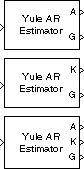# Yule-Walker AR Estimator

Compute estimate of autoregressive (AR) model parameters using Yule-Walker method

## Library

Estimation / Parametric Estimation

`dspparest3`

•## Description

The Yule-Walker AR Estimator block uses the Yule-Walker AR method, also called the autocorrelation method, to fit an autoregressive (AR) model to the windowed input data by minimizing the forward prediction error in the least squares sense. This formulation leads to the Yule-Walker equations, which are solved by the Levinson-Durbin recursion. Block outputs are always nonsingular.

The Yule-Walker AR Estimator block can output the AR model coefficients as polynomial coefficients, reflection coefficients, or both. The input can be a row vector, a column vector, or an unoriented vector which is assumed to be the output of an AR system driven by white noise. The block accepts matrices, and treats each column of the matrix as a channel. If the input is a row vector of length N, the input is treated as N different channels. If the input is an unoriented vector, the input is treated as a single channel. The block computes the normalized estimate of the AR system parameters, A(z), independently for each successive input frame.

`$H\left(z\right)=\frac{\sqrt{G}}{A\left(z\right)}=\frac{\sqrt{G}}{1+a\left(2\right){z}^{-1}+\dots +a\left(p+1\right){z}^{-p}}$`

When you select Inherit estimation order from input dimensions, the order p of the all-pole model is one less than the length of each input channel. Otherwise, the order is the value specified by the Estimation order parameter. To guarantee a valid output, you must set the Estimation order parameter to be a scalar less than or equal to half the input channel length. The Yule-Walker AR Estimator and Burg AR Estimator blocks return similar results for large frame sizes.

When Output(s) is set to `A`, port A is enabled. For each channel, port A outputs a column of length p+1 that contains the normalized estimate of the AR model coefficients in descending powers of z

```[1 a(2) ... a(p+1)] ```

When Output(s) is set to `K`, port K is enabled. For each channel, port K outputs a length-p column whose elements are the AR model reflection coefficients. When Output(s) is set to `A and K`, both port A and K are enabled, and each port outputs the respective AR model coefficients for each channel.

The square of the model gain, G, is provided at port G. G is a scalar for each channel.

See the Burg AR Estimator block reference page for a comparison of the Burg AR Estimator, Covariance AR Estimator, Modified Covariance AR Estimator, and Yule-Walker AR Estimator blocks.

## Parameters

Output(s)

The type of AR model coefficients output by the block. The block can output polynomial coefficients (`A`), reflection coefficients (`K`), or both (```A and K```).

Inherit estimation order from input dimensions

When selected, sets the estimation order p to one less than the length of each input channel.

Estimation order

The order of the AR model, p. This parameter is enabled when you do not select Inherit estimation order from input dimensions.

## References

Kay, S. M. Modern Spectral Estimation: Theory and Application. Englewood Cliffs, NJ: Prentice-Hall, 1988.

Marple, S. L., Jr., Digital Spectral Analysis with Applications. Englewood Cliffs, NJ: Prentice-Hall, 1987.

## Supported Data Types

PortSupported Data Types

Input

• Double-precision floating point

• Single-precision floating point

A

• Double-precision floating point

• Single-precision floating point

K

• Double-precision floating point

• Single-precision floating point

G

• Double-precision floating point

• Single-precision floating point

 Burg AR Estimator DSP System Toolbox Covariance AR Estimator DSP System Toolbox Modified Covariance AR Estimator DSP System Toolbox Yule-Walker Method DSP System Toolbox `aryule` Signal Processing Toolbox